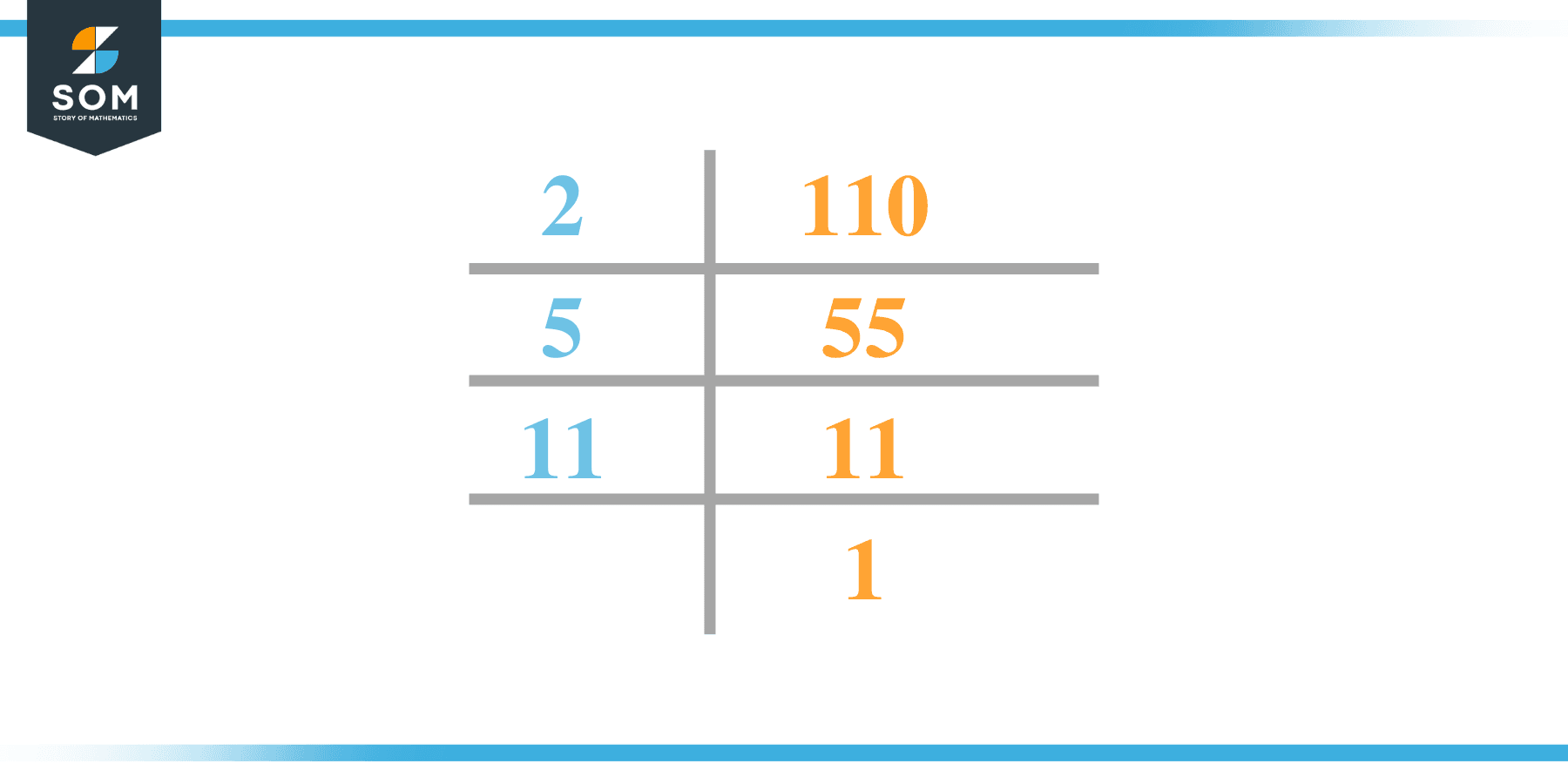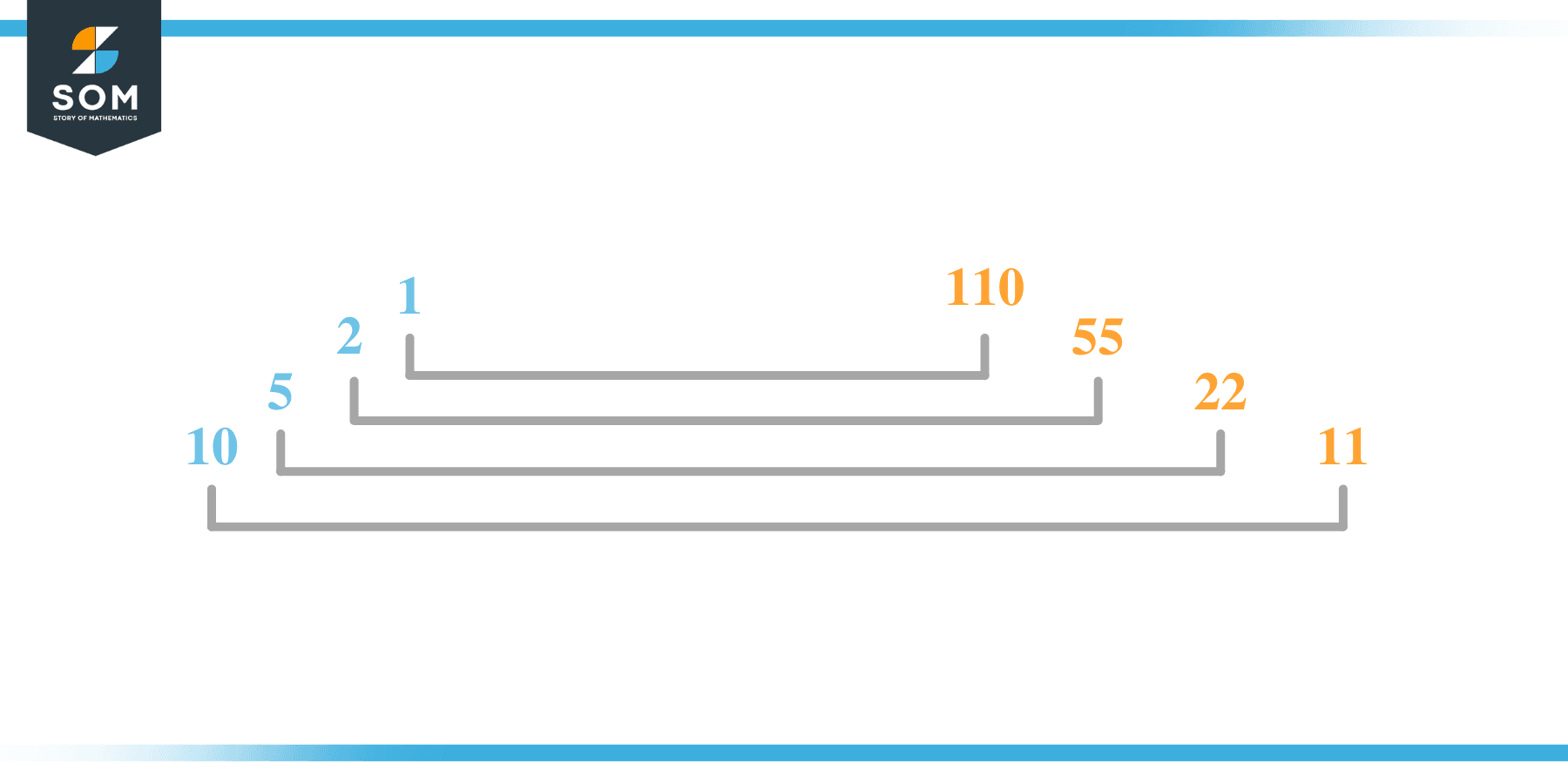# Factors of 110: Prime Factorization, Methods, and Examples

The factors of 110 are the numbers that produce zero as a reminder when 110 is divided by such numbers. These when, when they act as the divisors, also produce a whole number quotient.In such a case, both the divisors and the whole number quotients are considered as the factors of 110. In this article, we will dive into the depth of these factors and how to determine them.

### Factors of 110

Here are the factors of number 110.

Factors of 110: 1, 2, 5, 10, 11, 22, 55, 110

### Negative Factors of 110

The negative factors of 110 are similar to its positive factors, just with a negative sign.

Negative Factors of 110: -1, -2, -5, -10, -11, -22, -55 and -110

### Prime Factorization of 110

The prime factorization of 110 is the way of expressing its prime factors in the form of a product.

Prime Factorization: 2 x 5 x 11

In this article, we will learn about the factors of 110 and how to find them using various techniques such as upside-down division, prime factorization, and factor tree.

## What Are the Factors of 110?

The factors of 110 are 1, 2, 5, 10, 11, 22, 55, and 110. All of these numbers are the factors as they do not leave any remainder when divided by 110.

The factors of 110 are classified as prime numbers and composite numbers. The prime factors of the number 110 can be determined using the technique of prime factorization.

## How To Find the Factors of 110?

You can find the factors of 110 by using the rules of divisibility. The rule of divisibility states that any number when divided by any other natural number then it is said to be divisible by the number if the quotient is the whole number and the resulting remainder is zero.

To find the factors of 110, create a list containing the numbers that are exactly divisible by 110 with zero remainders. One important thing to note is that 1 and 110 are the 110’s factors as every natural number has 1 and the number itself as its factor.

1 is also called the universal factor of every number. The factors of 110 are determined as follows:

$\dfrac{110}{1} = 110$

$\dfrac{110}{2} = 55$

$\dfrac{110}{5} = 22$

$\dfrac{110}{10} = 11$

$\dfrac{110}{11} = 10$

$\dfrac{110}{22} = 5$

$\dfrac{110}{55} = 2$

$\dfrac{110}{110} = 1$

Therefore, 1, 2, 5, 10, 11, 22, 55, and 110 are the factors of 110.

### Total Number of Factors of 110

For 110 there are 8 positive factors and 8 negative ones. So in total, there are 16 factors of 110.

To find the total number of factors of the given number, follow the procedure mentioned below:

1. Find the factorization of the given number.
2. Demonstrate the prime factorization of the number in the form of exponent form.
3. Add 1 to each of the exponents of the prime factor.
4. Now, multiply the resulting exponents together. This obtained product is equivalent to the total number of factors of the given number.

By following this procedure the total number of factors of 110 is given as:

Factorization of 110 is 1 x 2 x 5 x 11.

The exponent of all these factors is 1.

Adding 1 to each and multiplying them together results in 16.

Therefore, the total number of factors of 110 is 16, where 8 are positive factors and 8 are negative factors.

### Important Notes

Here are some important points that must be considered while finding the factors of any given number:

• The factor of any given number must be a whole number.
• The factors of the number cannot be in the form of decimals or fractions.
• Factors can be positive as well as negative.
• Negative factors are the additive inverse of the positive factors of a given number.
• The factor of a number cannot be greater than that number.
• Every even number has 2 as its prime factor which is the smallest prime factor.

## Factors of 110 by Prime FactorizationThe number 110 is composite. Prime factorization is a useful technique for finding the number’s prime factors and expressing the number as the product of its prime factors.

Before finding the factors of 110 using prime factorization, let us find out what prime factors are. Prime factors are the factors of any given number that are only divisible by 1 and themselves.

To start the prime factorization of 110, start dividing by its smallest prime factor. First, determine that the given number is either even or odd. If it is an even number, then 2 will be the smallest prime factor.

Continue splitting the quotient obtained until 1 is received as the quotient. The prime factorization of 110 can be expressed as:

110 = 2 x 5 x 11

## Factors of 110 in PairsThe factor pairs are the duplet of numbers that when multiplied together result in the factorized number. Depending upon the total number of factors of the given numbers, factor pairs can be more than one.

For 110, the factor pairs can be found as:

1 x 110 = 110

2 x 55 = 110

5 x 22 = 110

10 x 11 = 110

The possible factor pairs of 110 are given as (1, 110), (2, 55), (5, 22), and (10, 11).

All these numbers in pairs, when multiplied, give 110 as the product.

The negative factor pairs of 110 are given as:

-1 x -110 = 110

-2 x -55 = 110

-5 x -22 = 110

-10 x -11 = 110

It is important to note that in negative factor pairs, the minus sign has been multiplied by the minus sign due to which the resulting product is the original positive number. Therefore, -1, -2, -5, -10, -11, -22, -55, and -110 are called negative factors of 110.

The list of all the factors of 110 including positive as well as negative numbers is given below.

Factor list of 110: 1, -1, 2, -2, 5, -5, 10, -10, 11, -11, 22, -22, 55, -55, 110, and -110

## Factors of 110 Solved Examples

To better understand the concept of factors, let’s solve some examples.

### Example 1

How many factors of 110 are there?

### Solution

The total number of Factors of 110 is 8.

Factors of 110 are 1, 2, 5, 10, 11, 22, 55, and 110.

### Example 2

Find the factors of 110 using prime factorization.

### Solution

The prime factorization of 110 is given as:

110 $\div$ 2 = 55

55 $\div$ 5 = 11

11 $\div$ 11 = 1

So the prime factorization of 110 can be written as:

2 x 5 x 11 = 110